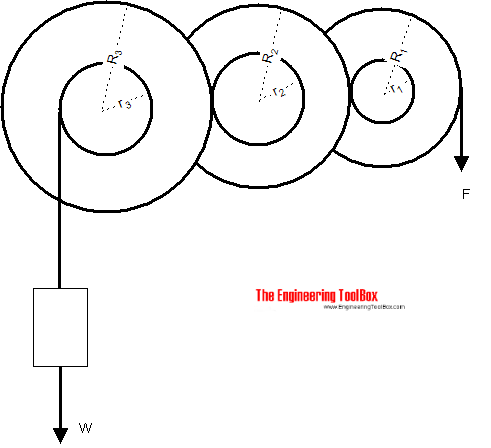Engineering ToolBox - Resources, Tools and Basic Information for Engineering and Design of Technical Applications!

# Gears

## Gears effort force vs. load force.

A gear is a mechanical device that transmits rotary motion by changing the magnitude and line of action of the effort force.The effort force can be expressed as

F = W (r1 r2 .. rn) / (R1 R2 .. Rn)                                     (1)

where

F = effort force (N, lbf)

W = load force or weight (N, lbf)

r = gear wheel inside diameter (m, in)

R = gear wheel outside diameter (m, in)

### Example - Gear Transmission and Effort Force

The effort force in a gear transmission with three wheels:

• wheel 1 : r1 = 0.05, R1 = 0.12
• wheel 2 : r2 = 0.06, R2 = 0.13
• wheel 3 : r3 = 0.07, R3 = 0.14

and a weight load of 1000 N can be calculated as

F = W (r1 r2 rn) / (R1 R2 Rn)

= (1000 N) ((0.05 m) (0.06 m) (0.07 m)) / ((0.12 m) (0.13 m) (0.14 m))

= 96 N

= 0.1 kN

## Related Topics

• ### Mechanics

Forces, acceleration, displacement, vectors, motion, momentum, energy of objects and more.

## Related Documents

• ### Belt Transmissions - Speed and Length of Belts

Calculate length and speed of belt and belt gearing.
• ### Belts - Pulley Diameters vs. Speed

The pulley laws - driver and driven - diameter and rpm
• ### Conn-Rod Mechanism

The connecting rod mechanism.
• ### Efficiency of Small Machine Elements

Friction and efficiency in bearings and roller chains.
• ### Force

Newton's third law - force vs. mass and acceleration.
• ### Force Ratio

The force ratio is the load force versus the effort force.
• ### Gear Reducing Formulas

Output torque, speed and horsepower with gears.
• ### Gear Trains - Bicycle Gearing Calculator

Gear train transmissions - bicycle gearing.
• ### Mass vs. Weight

Mass vs. weight - the Gravity Force.
• ### Toggle Joint

A toggle joint mechanism can be used to multiply force.

## Engineering ToolBox - SketchUp Extension - Online 3D modeling!

Add standard and customized parametric components - like flange beams, lumbers, piping, stairs and more - to your Sketchup model with the Engineering ToolBox - SketchUp Extension - enabled for use with older versions of the amazing SketchUp Make and the newer "up to date" SketchUp Pro . Add the Engineering ToolBox extension to your SketchUp Make/Pro from the Extension Warehouse !

We don't collect information from our users. More about

## Citation

• The Engineering ToolBox (2008). Gears. [online] Available at: https://www.engineeringtoolbox.com/gears-d_1307.html [Accessed Day Month Year].

Modify the access date according your visit.

9.19.12